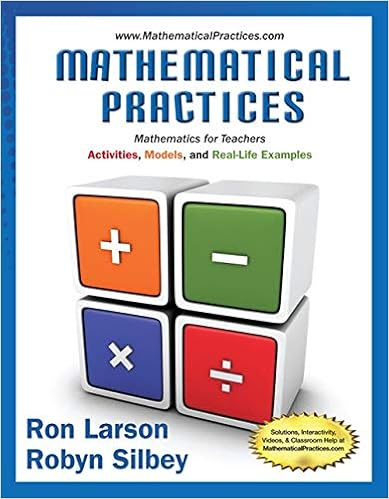# Franklin measured and labeled the angles of a

• Homework Help
• GustavoMX
• 3
• 97% (33) 32 out of 33 people found this document helpful

This preview shows page 2 - 3 out of 3 pages.

##### We have textbook solutions for you!
The document you are viewing contains questions related to this textbook.The document you are viewing contains questions related to this textbook.
Chapter 10 / Exercise 31
Mathematical Practices, Mathematics for Teachers: Activities, Models, and Real-Life Examples
LarsonExpert Verified
3. Franklin measured and labeled the angles of a triangle as shown. Jennifer says that at least one of his measures is incorrect. Explain in at least two different ways how Jennifer knows that this is true. 4. Joey says that triangle XYZ is obtuse. Robin disagrees because there are more acute angles then there are obtuse angles therefore it must be acute. Is either one of them correct? Explain your reasoning. 5. Compare and contrast the perpendicular bisectors and angle bisectors of a triangle. How are they alike? How are they different? 6. Compare and contrast the perpendicular bisectors, medians, and altitudes of a triangle. How are they alike? How are they different?
##### We have textbook solutions for you!
The document you are viewing contains questions related to this textbook.The document you are viewing contains questions related to this textbook.
Chapter 10 / Exercise 31
Mathematical Practices, Mathematics for Teachers: Activities, Models, and Real-Life Examples
LarsonExpert Verified
•••